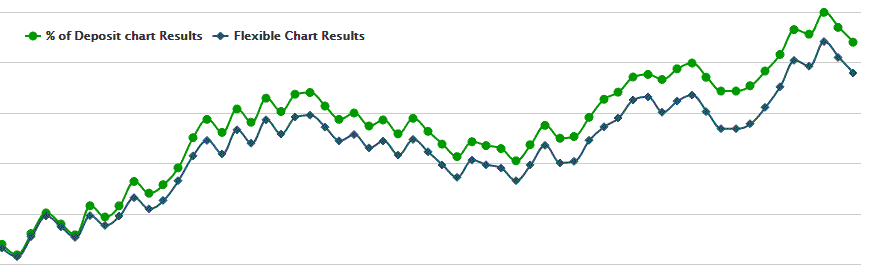Successful (profitable) trading is mostly an appropriate management of both profits and losses (risk and reward). Trading lot size is the most important parameter within a risk / money management scope. How many lots shall you open? How much shall you risk for each trade? These and many other questions are the questions any investor faces in the beginning of a trading career. If you do not make a decision before you enter a market, you will definitely lose most of your capital some day.

Signalator uses various trading approaches and trading signals. To maximize results and profits, consider the following page with explanation and examples of possible trade lot types. As most customers use automated trading, all these options are integrated within an autotrader (automated execution of trades).

FIXED LOT Fixed lot size regardless previous results and / or account size.
FLEXIBLE Calculates an amount of lot depending on a current level of an account.
PERCENTAGE OF A DEPOSIT Open as many lots to limit a risk by a chosen percentage of deposit.

There are two basic things we will be describing: a lot type and a lot size. Lot type is a way you or an autotrader calculates a required size of an opening position. Lot size is an actually an amount you buy or sell. The first is used for calculation of the second. Currently we offer the three lot types (options for calculating a lot size): Fixed, Flexible and % of Deposit (percentage of deposit). Each has pros and cons. Moreover, under different trading circumstances, you may use different lot type options. Please note: the following page only describes the possible types of lot sizing. If you require an information on risk / money management, please use the recommended deposits page or alternatively visit the Signalator Investment Calculator for more sophisticated approach.

## FIXED LOT TYPE

Calculation: basically there is no calculation at all. You simply put a desired amount of lot (e.g. 0.13).

Settings: Choose "Fixed" with the lot type option. Put value of a lot in Lot size field => 0.15

Strong sides: trade the same level of lots. If trading results are within average results, you will be getting an expected level of profit.

Weak sides: if you experience a loss period, your account will be vanishing rapidly. On the contrary, if you face a good trading period, you won't get as much as you could.

OVERALL: THE LEAST EFFICIENT TYPE OF TRADING

### FLEXIBLE LOT TYPE

Flexible lot is an advanced way of a fixed lot with elements of risk management similar to the % of Deposit lot type. Position size is calculated for every new trade. The calculation is based on the account equity value. The higher equity you have the more lots you open and vice versa. Once you decided how many lots you will be opening for your current equity size, you define two parameters: lot size and flexible deposit level. Deposit level will be used to calculate the amount of lots you shall open. In other words autotrader will be opening as many lots as required based on a simple rule: open Y lots for every X deposit level.

The calculation is very simple: take the account equity (account balance + currently open trades result) divide it by the flexible deposit level and multiply by lot size. (EQUITY / FLEX DEPOSIT ) * LOT SIZE = OPEN AMOUNT. For illustration purposes, we will use a deposit of \$5,000 with \$2,500 flexible deposit level and 0.1 lot for every \$2,500. Settings are: Flexible deposit level = \$2,500, lot size = 0.1. Every time a new trade is opened, we will take an account value divide it by flexible lot size and then multiply by lot size. In our example, it will be \$5,000 / \$2,500 =>2, then multiply by 0.1 =>0.2. So we have to open 0.2 lot for \$5,000 equity. Let us assume, an account has grown to \$6,250 value. The calculation is the same: (\$6,250 / \$2,500 ) * 0.1 = 2.5 * 0.1 => 0.25 lots. If account to fall in its value, e.g. now it is \$3,750 then we will have 1.5 * 0.1 = 0.15 lots. This calculation is made for every new trade an hence the amounts of lot will be changing smoothly.

The following image demonstrates the process of lot calculation for every new trade. Flexible lot and flexible lot sizes are constant values. As account equity changes, the lot size for the next trade is affected. The higher is the account value, the more lots we open and vice versa.

Further, you will find an example of Flexible Lot trading used for the Signalator Plus from 1st of January 2016 with \$2,500 flexible deposit and 0.1 flexible lot. You may check these results on the Signalator Investment Calculator.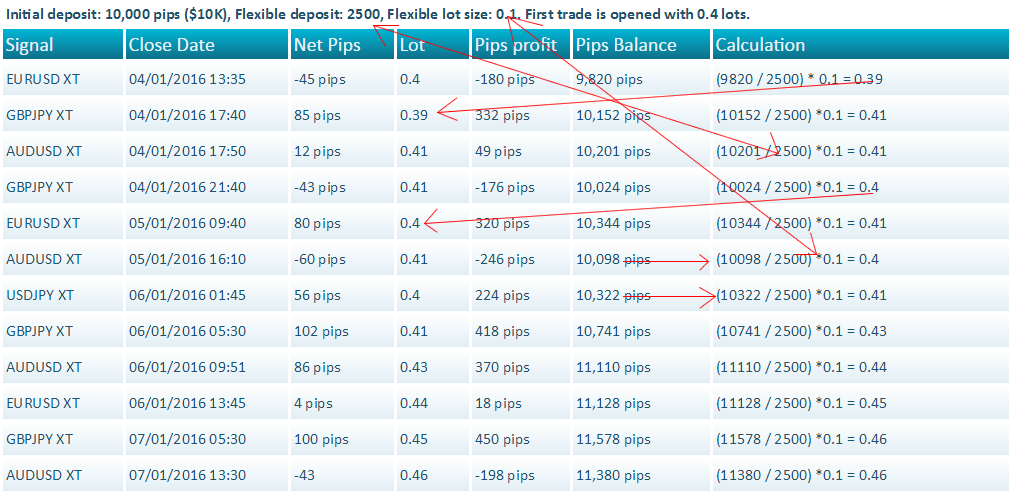Calculation: ACCOUNT EQUITY / FLEXIBLE DEPOSIT LEVEL * LOT SIZE => (e.g. (\$5,000 / \$2,500) * 0.1 = 0.2 lots).

Settings :Choose "Flexible" within the lot type; Put Flexible deposit level into the Flexible Deposit field and lot size to Lot Size field (e.g. Flex Deposit = 2500, Flex lot = 0.1).

Strong sides: profits increase with account growth; losses reduces as account equity drops.

Weak sides: risk level for each trade is different and depends on level of a SL.eralHE MOST EFFICIENT LOT TYPE FOR SIGNALATOR SIGNALS

#### % OF DEPOSIT RISK LOT TYPE

This type of lot is widely used among the forex traders. Every new trade will risk a certain fixed amount of an equity balance (e.g. 2%). This is the reason why traders prefer to use this type of lot. You control the maximum level of risk for every trade based on a percentage of your deposit. So profits and losses will have results relative to an account equity. The more funds you have the more profit you will be getting (and losses as well). If an account value goes down, both losses and profits will be small as well. This kind of trading make trading very flexible.

The calculation involves several steps to be performed: 1) Define the maximum loss for a trade based on the chosen % of risk (e.g. 3%), then the maximum loss for a trade should be 3% of equity. This value is the value of SL in an account currency; 2) The general calculation formula is as follows: RISK IN % * EQUITY => MAX LOSS IN CURRENCY; THEN WE GET THE LEVEL OF SL => SL AND VALUE IN CURRENCY OF AN ACCOUNT FOR EACH PIP. MAX LOSS IN CURRENCY / (VALUE OF PIP IN ACCOUNT CURRENCY * SL LEVEL) => LOT SIZE

Further, you will find an example of Flexible Lot trading used for the Signalator Plus from 1st of January 2016 with \$2,500 flexible deposit and 0.1 flexible lot. You may check these results on the Signalator Investment Calculator.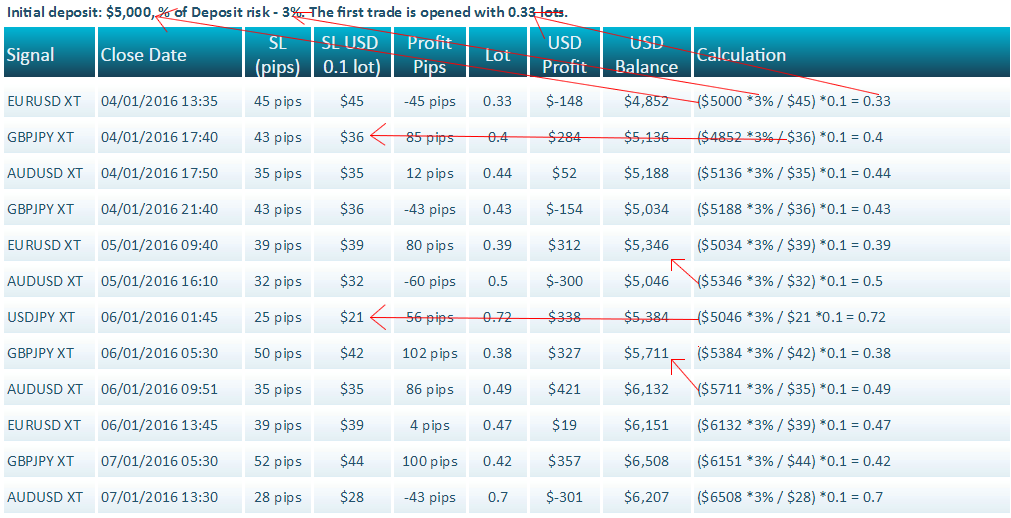Calculation: GET THE SL LEVEL IN PIPS AND VALUE OF A PIP IN AN ACCOUNT CURRENCY; GET ACCOUNT CURRENCY MONEY VALUE OF SL; DIVIDE SL VALUE IN CURRENCY BY MULTIPLIED SL IN PIPS AND VALUE OF A PIP

Settings: Choose the "% of Deposit" with the lot type option. Set a desired level of lot without "%" sign (e.g. 3).

Strong sides: in every trade you define the same risk level of your deposit in percentage to your deposit; losses are flexible-> the higher is the account value, the higher losses you may have and if your account is reduced your losses will be lower in terms of money value.

Weak sides: if a trade several signals with different SL levels and hence various mathematical expectations, you will be getting more losses compared to flexible and fixed lots.

Overall:ONE OF THE MOST POPULAR LOT SIZE OPTIONS

##### Different lot type trading comparison

The best way to understand which type of lot is the most appropriate for or which one generates more profits, less losses is to compare these types side by side. Further you will find a series of reports based on the Signalator Investment Calculator calculations. You may perform an unlimited number of analysis report to find out which type suits you best. All trades are the results of signals we sent to our clients. We will compare the two most popular trading types: Flexible Lot and % of Deposit.

Example 1. Signalator Plus from January 2016. The settings for lot types are configured to have the same level of Drawdown for both types.

Initial Equity: \$5,000; % of Deposit: 3%; Flexible deposit: \$3,000, Flexible lot: 0.1.The following example is for the Signalator Plus signals set traded from the 1st of January 2016 to the end of July shows Flexible lot made net 128% profit whilst % of Deposit generated 95% of profit. However, the drawdown level was the same. It means, with the same level of risk, the Flexible Lot size performs better.

As you can see, in this case the Flexible Lot trading type was approximately 35% more profitable.

Chart 1. The following chart provides a visual comparison of these two lot types.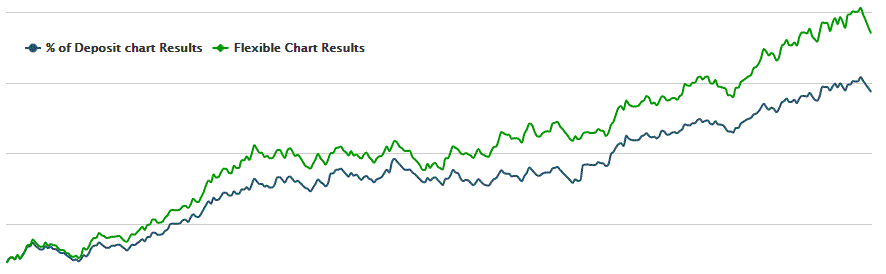Example 2. EURUSD XT, USDJPY XT and Gold. The settings for lot types are configured to have the same level of Net Profit for both types.

Initial Equity: \$5,000; % of Deposit: 2%; Flexible deposit: \$2,000, Flexible lot: 0.11.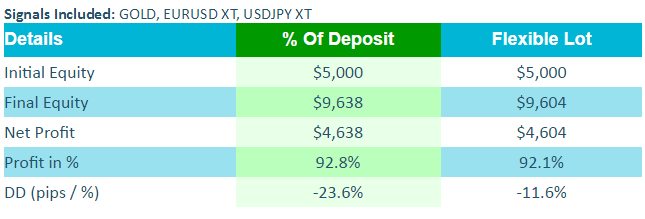The following example is for EURUSD XT, USDJPY XT and Gold signals traded from 1st of January to the end of July. Both types provide the same level of profit, however, % of Deposit has twice as big level of Drawdown compared to Flexible lot.

In terms of risk / reward, Flexible Lot seems to be more attractive.

Chart 2. As you can see from the chart, Flexible Type provides a smooth equity curve without sharp ups and downs, whereas % of Deposit is very volatile.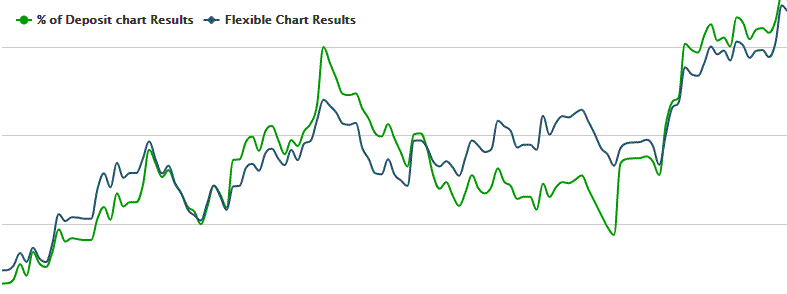Example 3. GBPJPY XT. The settings for lot types are configured to have the same level of Net Profit for both types.

Initial Equity: \$5,000; % of Deposit: 2%; Flexible deposit: \$2,200, Flexible lot: 0.1.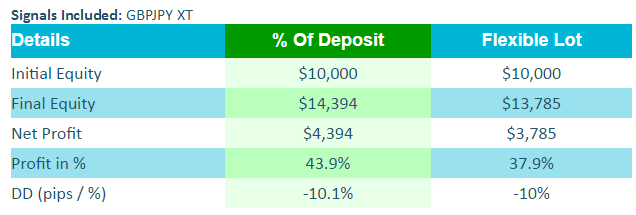The following example is for the GBPJPY XT signal traded from 1st of January to the end of July. Both types provide the same level of drawdown, but % of Deposit made 6% more profits than a Flexible lot trading. This is an example of a trading when % of Deposit is more profitable.

Chart 3. As you can see from the chart, trading with % of Deposit for this signal provided more profits for any period of time.